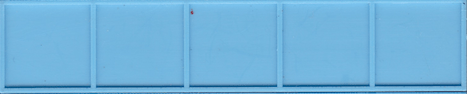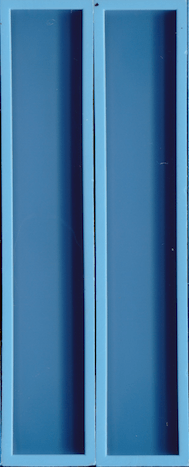# zero pair models for multiplying positives and negatives together

ABOUT THE LANGUAGE AND MODELS

There are multiple ways to verbalize and model the concept of multiplying positive and negatives together. This page shows one way the symbols can be read and the blocks can be used to model the language. It also contains important Mortensen more than Math language and models. Give students time to practice and play using the language and building the models with blocks.

MORTENSEN MORE THAN MATH LANGUAGE AND MODELS

Numbers have FIRST NAMES – HOW MANY and LAST NAMES – WHAT KIND.

POSITIVE means HAVE and NEGATIVE means OWE.

The segmented side of a bar is considered POSITIVE thus a five bar with its segmented side up means we HAVE FIVE of the UNITS KIND or +5 units.The hollow side of a bar is considered NEGATIVE thus a 5 bar with its hollow side up means we OWE FIVE of the UNITS KIND or 5 units.If we HAVE and OWE the same amount we have ZERO or a ZERO PAIR+5 units and 5 units add to ZERO and so are a ZERO PAIR. This ZERO PAIR can be modelled with two five bars, one with its segmented side up and one with its hollow side up, placed lengthwise beside each other.MULTIPLICATION OF POSITIVES AND NEGATIVES WITH ZERO PAIR LANGUAGE AND MODELS

PROBLEM ONE
A) (+2)(+5) reads from left to right HAVE TWO POSITIVE FIVES or ADD TWO POSITIVE FIVES. We take two five bars from the box and place them segmented side up which gives us the answer +10.B) (+5)(+2) reads from left to right HAVE FIVE POSITIVE TWOS or ADD FIVE POSITIVE TWOS. We take five two bars from the box and place them segmented side up which gives us the answer +10.PROBLEM TWO
A) (+2)(5) reads from left to right HAVE TWO NEGATIVE FIVES or ADD TWO NEGATIVE FIVES. We take two five bars from the box and place them hollow side up which gives us the answer 10.B) (+5)(2) reads from left to right HAVE FIVE NEGATIVE TWOS or ADD FIVE NEGATIVE TWOS. We take five two bars from the box and place them hollow side up which gives us the answer 10.PROBLEM THREE
Problem 2 and 3 are similar and have the same answers however they are written, read, and modelled differently with the ZERO PAIR method.

A) (5)(+2) reads from left to right OWE FIVE POSITIVE TWOS or SUBTRACT FIVE POSITIVE TWOS but how can we subtract from nothing? We can build ZERO PAIRS out of five two bars segmented side up and five two bars hollow side up. This allows us to remove five segmented two bars which leaves us with five hollow two bars and gives us the answer 10.B) (2)(+5) reads from left to right OWE TWO POSITIVE FIVES or SUBTRACT TWO POSITIVE FIVES but how can we subtract from nothing? We can build ZERO PAIRS with two five bars segmented side up and two five bars hollow side up. This allows us to remove two segmented five bars which leaves two hollow five bars and gives us the answer 10.PROBLEM FOUR

A) (2)(5) reads from left to right OWE TWO NEGATIVE FIVES or SUBTRACT TWO NEGATIVE FIVES but how can we subtract from nothing? We can build ZERO PAIRS with two five bars segmented side up and two five bars hollow side up. This allows us to remove two hollow five bars which leaves two segmented five bars and gives us the answer +10.B) (5)(2) tells us that we OWE FIVE NEGATIVE TWOS or must SUBTRACT FIVE NEGATIVE TWOS but how can we do this when we have nothing to subtract from? We can build ZERO PAIRS with five two bars segmented side up and five two bars hollow side up. This allows us to remove five hollow two bars which leaves five segmented two bars and gives us the answer +10.FIELD OF ZEROS
This video shows how to multiply positives and negatives using a field of ZEROS which is essentially the same as the concept being modelled above.

FUN AT THE BEACH
Watch this innovative video posted by Arithmophobia No More to see a fun beach activity you can do with your child to help them learn about ZERO PAIRS.